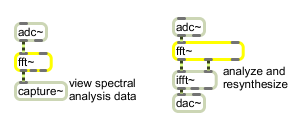# fft~

Fast Fourier transform

## Description

fft~ performs a Fast Fourier transform on any incoming signal and outputs the real and imaginary parts of that transform as well as a synchronization signal.

## Arguments

Name Type Opt Description
number-of-FFT-samples int opt The first argument specifies the number of points (samples) in the FFT. It must be a power of two. The default number of points is 512. The second argument specifies the number of samples between successive FFTs. This must be at least the number of points, and must also be a power of two. The default interval is 512. The third argument specifies the offset into the interval where the FFT will start. This must either be 0 or a multiple of the signal vector size. fft~ will correct bad arguments, but if you change the signal vector size after creating an fft~ and the offset is no longer a multiple of the vector size, the fft~ will not operate when signal processing is turned on.

## Messages

 signal In left inlet: The real part of a complex signal that will be transformed. In right inlet: The imaginary part of a complex signal that will be transformed. If signals are connected only to the left inlet and left outlet, a real FFT (fast Fourier transform) will be performed. Otherwise, a complex FFT will be performed.

## Output

signal: Out left outlet: The real part of the Fourier transform of the input. The output begins after all the points of the input have been received.

Out middle outlet: The imaginary part of the Fourier transform of the input. The output begins after all the points of the input have been received.

Out right outlet: A sync signal that ramps from 0 to the number of points minus 1 over the period in which the FFT output occurs. You can use this signal as an input to the index~ object to perform calculations in the frequency domain. When the FFT is not being sent out (in the case where the interval is larger than the number of points), the sync signal is 0.

## ExamplesFast Fourier transform of an audio signal

Name Description
cartopol Cartesian to Polar coordinate conversion
cartopol~ Signal Cartesian to Polar coordinate conversion
fftin~ Input for a patcher loaded by pfft~